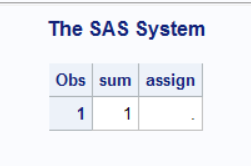## Issue with dataset + operator

``````data s2;
retain sum;
assign = sum;
sum + 1;
proc print; run;``````*Anyone knows why here:*

assign=. whereas

sum=1
because when we apply the + operator with any missing value the final result should be. (missing)

1 ACCEPTED SOLUTION

Accepted Solutions

## Re: Issue with dataset + operator

If you use a variable as the target in a SUM statement then the variable will be retained and it will default to initialize to zero , UNLESS YOU TELL TO INITIALIZE TO SOMETHING ELSE.

Remove the RETAIN statement.  Of tell it to initialize to zero instead of missing.

```19   data s2;
20   retain sum;
21   assign = sum;
22   sum + 1;
23   put (_all_) (=);
24   run;

sum=1 assign=.
NOTE: The data set WORK.S2 has 1 observations and 2 variables.
NOTE: DATA statement used (Total process time):
real time           0.03 seconds
cpu time            0.04 seconds

25   data s2;
26   assign = sum;
27   sum + 1;
28   put (_all_) (=);
29   run;

assign=0 sum=1
NOTE: The data set WORK.S2 has 1 observations and 2 variables.
NOTE: DATA statement used (Total process time):
real time           0.01 seconds
cpu time            0.03 seconds

30   data s2;
31   retain sum 100;
32   assign = sum;
33   sum + 1;
34   put (_all_) (=);
35   run;

sum=101 assign=100
NOTE: The data set WORK.S2 has 1 observations and 2 variables.
NOTE: DATA statement used (Total process time):
real time           0.00 seconds
cpu time            0.00 seconds
```
4 REPLIES 4

## Re: Issue with dataset + operator

The SUM statement documentation says the variable (in this case SUM) is initialized to zero. So sum+1 is not missing.

--
Paige Miller

## Re: Issue with dataset + operator

There are three "sum" methods.
Sum Operator
Sum Statement
Sum Function

They each works slightly differently.

## Re: Issue with dataset + operator

If you use a variable as the target in a SUM statement then the variable will be retained and it will default to initialize to zero , UNLESS YOU TELL TO INITIALIZE TO SOMETHING ELSE.

Remove the RETAIN statement.  Of tell it to initialize to zero instead of missing.

```19   data s2;
20   retain sum;
21   assign = sum;
22   sum + 1;
23   put (_all_) (=);
24   run;

sum=1 assign=.
NOTE: The data set WORK.S2 has 1 observations and 2 variables.
NOTE: DATA statement used (Total process time):
real time           0.03 seconds
cpu time            0.04 seconds

25   data s2;
26   assign = sum;
27   sum + 1;
28   put (_all_) (=);
29   run;

assign=0 sum=1
NOTE: The data set WORK.S2 has 1 observations and 2 variables.
NOTE: DATA statement used (Total process time):
real time           0.01 seconds
cpu time            0.03 seconds

30   data s2;
31   retain sum 100;
32   assign = sum;
33   sum + 1;
34   put (_all_) (=);
35   run;

sum=101 assign=100
NOTE: The data set WORK.S2 has 1 observations and 2 variables.
NOTE: DATA statement used (Total process time):
real time           0.00 seconds
cpu time            0.00 seconds
```
Discussion stats
• 4 replies
• 219 views
• 2 likes
• 4 in conversation